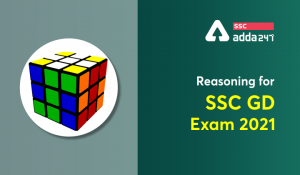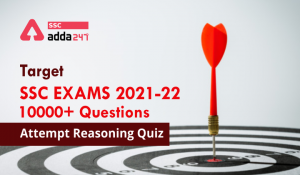Latest SSC jobs   »   Reasoning Quiz For SSC Exams :-...

# Reasoning Quiz For SSC Exams :- 25th Sep.

Dear aspirants,

SSC CGL 2019 Exam notification is on its way to once again raise the bar of competition. All the four sections bag impeccable practice when it comes to SSC Exams. Considering the same, at ADDA247 we are prompted to make Daily Reasoning Quiz accessible to you, aiming at a cut above preparation of latest exam pattern based questions. You are asked to practice Daily Reasoning Quiz at SSCADDA which encapsulates the exam like environment facilitated with extraordinary content. To make headway in upcoming SSC CGL Exams, go the extra mile with our Daily Reasoning Quiz.

Direction(1-10) : Following series are given below with one term missing. Choose the correct alternative from the given ones that will complete the series.
एक श्रंखला दी गई है जिसमें एक टर्म अज्ञात हैं आपको दिए गए विकल्पों में से उस विकल्प का चयन करना है जो इस श्रंखला को पूरा करेगा?
Q1. 2, 5, 9, 19, 37 ?
(a) 73
(b) 75
(c) 76
(d) 78

S1. Ans. (b)
Sol. Clearly, we have: 2 x 2 + 1= 5, 5 x 2 -1 = 9, 9 x 2 + 1 = 19, 19 x 2 -1 = 37,
So, missing term = 37 x 2 + 1 =75.

Q2. 22, 24, 28, ?, 52, 84
(a) 36
(b) 38
(c) 42
(d) 46

S2. Ans. (a)
Sol. The pattern is +2, +4, +8, +16
So, missing term = 28 + 8 = 36.

Q3. 1, 4, 27, 16, ?, 36, 343
(a) 25
(b) 87
(c) 120
(d) 125

S3. Ans. (d)
Sol. Clearly, the given the series consists of cubes of odd numbers and squares of even numbers, I. e, 13, 23, 33, 43, ….
So, missing term = 53 = 125.

Q4. 1/2, 3/4, 5/8, 7/16, ?
(a) 9/32
(b) 10/17
(c) 11/34
(d) 12/35

S4. Ans. (a)
Sol. Clearly, the numerators of the fractions in the given sequence form the series 1, 3, 5, 7, in which each term is obtained by adding 2 to the previous term.The denominators of the fractions form the series 2, 4, 8, 16, i.e. 21, 22, 23, 24.So, the numerator of the fractions will be (7 +2) i.e. 9 and the denominator will be 25 i.e. 32.
Thus, the next term is 9/32.

Q5. 1, 9, 25, 49, ?, 121
(a) 64
(b) 81
(c) 91
(d) 100

S5. Ans. (b)
Sol. The given series consists of squares of consecutive odd numbers i.e. 12, 32, 52,72,…… So, missing term = 92 =81

Q6. B, D, F, I, L, P, ?
(a) R
(b) S
(c) T
(d) U

S6. Ans. (c)
Sol. B(+2)→D (+2)→ (+3)→ (+3)→ (+4)→P (+4)→T

Q7. U,B,I,P,W ,?
(a) D
(b) F
(c) Q
(d) U

S7. Ans. (a)
Sol. U (+7)→B (+7)→I (+7)→P (+7)→ (+7)→D

Q8. Z, ?, T, ?, N, ?, H, ?, B
(a) W,Q,K,E
(b) W,R,K,E
(c) X,Q,K,E
(d) X,R,K,E

S8. Ans. (a)
Sol. Z (-6)→T (-6)→N (-6)→ (-6)→B
Z (-3)→ (-3)→T (-3)→Q (-3)→N (-3)→K (-3)→H (-3)→ (-3)→B

Q9. a , d , c , f , ?, h , g , ? , i
(a) e, j
(b) e, k
(c) f, j
(d) j, e
S9. Ans. (a)
Sol. a (+3)→d (-1)→c (+3)→f (-1)→e (+3)→ (-1)→g (+3)→j (-1)→i.

Q10. A, I, P, V, A, E, ?
(a) E
(b) F
(c) G
(d) H

S10. Ans. (d)
Sol. A (+8)→I (+7)→P (+6)→V (+5)→A (+4)→E (+3)→H

#### Check SSC CGL Tier 1 Expected Cut-off

You May Also Like To Read :

#### Congratulations!General Awareness & Science Capsule PDFIncorrect details? Fill the form again here

General Awareness & Science Capsule PDF

Thank You, Your details have been submitted we will get back to you.

### TOPICS:

•Reasoning for SSC GD 2021: 30th November
•Reasoning for SSC GD 2021: 29th November
•Reasoning for SSC GD 2021: 08 October
•Target SSC Exams 2021-22 10000+ Question...
•Target SSC Exams 2021-22 10000+ Question...
•Reasoning-Quizzes : Target SSC Exams 202...